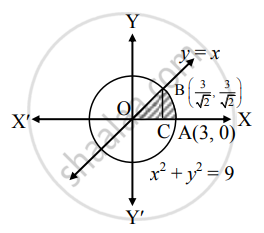# Find the area enclosed between the circle x2 + y2 = 9, along X-axis and the line x = y, lying in the first quadrant - Mathematics and Statistics

Sum

Find the area enclosed between the circle x2 + y2 = 9, along X-axis and the line x = y, lying in the first quadrant

#### Solution

Given equation of the circle is x2 + y2 = 9   .....(i)

and equation of the line is x = y  ......(ii)

From (i), we get

y2 = 9 −x2

∴ y = sqrt(9 - x^2)   .....(iii) .....[∵ In first quadrant, y > 0]

Substituting (ii) in (i), we get

x2 + x2 = 9

∴ 2x= 9

∴ x= 9/2

∴ x = 3/sqrt(2)    .....[∵ In first quadrant, x > 0]

When x = 3/sqrt(2), y = 3/sqrt(2)

∴ The point of intersection is "B"(3/sqrt(2), 3/sqrt(2)).Required area = area of the region OCABO

= area of the region OCBO + area of the region ABCA

= int_0^(3/sqrt(2)) x  "d"x + int_(3/(sqrt(2)))^3 sqrt(9 - x^2)  "d"x     .....[From (iii) and (ii)]

= 1/2[x^2]_0^(3/sqrt(2)) + [x/2 sqrt(9 - x^2) + 9/2  sin^-1 (x/3)]_(3/sqrt(2))^3

= 1/2[(3/sqrt(2))^2] - 0] + [3/2 sqrt(9 - 9) + 9/2  sin^-1  (1) - {3/(2sqrt(2))  sqrt(9 -  9/2) + 9/2  sin^-1  (1/sqrt(2))}]

= 9/4 + 9/2(pi/2) - 3/(2sqrt(2)) (3/sqrt(2)) - 9/2(pi/4)

= 9/4 + (9pi)/4 - 9/4 - (9pi)/8

= (9pi)/8 sq.units

Concept: Area Between Two Curves
Is there an error in this question or solution?